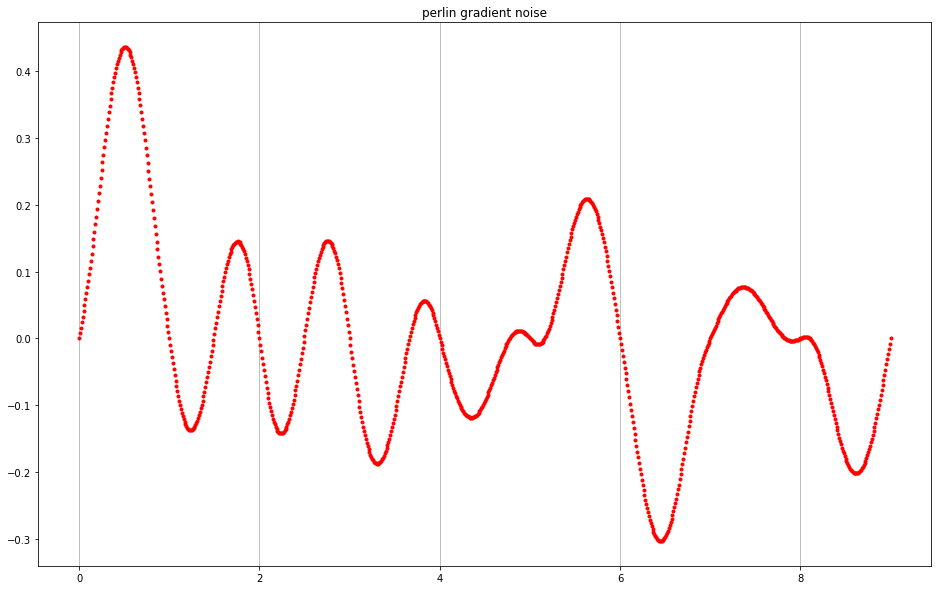In :
# demo of Perlin noise
# see blog post comparing pure randomness with value and gradient noise
# http://makeyourownalgorithmicart.blogspot.co.uk/2018/02/randomness-and-perlin-noise.html

In :
import numpy
import random
import math

import matplotlib.pyplot as plt
%matplotlib inline

In :
# polynomial smoothing function
# had zero derivataive at 0 and 1
# this one also has zero 2nd derivative

def blend(x):
return 6*x**5 - 15*x**4 + 10*x**3

In :
# how much data
how_much = 10

In :
# create a list of 2d vectors

random_vectors = []

for i in range(how_much):
angle = random.random() * math.tau;
x = math.cos(angle)
y = math.sin(angle)
random_vectors.append(numpy.array([x,y]))
pass

# show just the first ten
random_vectors[:10]

Out:
[array([ 0.79571533,  0.60567079]),
array([-0.94902087, -0.31521325]),
array([-0.97595829,  0.21795738]),
array([-0.98932984, -0.14569303]),
array([-0.53264581, -0.84633825]),
array([-0.15614321, -0.98773443]),
array([-0.86721564, -0.49793276]),
array([ 0.32239179, -0.94660633]),
array([ 0.0622803,  0.9980587]),
array([ 0.78341733,  0.62149601])]
In :
# traverse along x axis

x_values = []
noise_values = []

x = 0
while(x < (how_much-1)):
# find nearest vectors
below = math.floor(x)
above = below + 1

# calculate the dot products between the random vectors and the vector to x
dotproduct_1 = numpy.dot(random_vectors[below], numpy.array([x-below,0]))
dotproduct_2 = numpy.dot(random_vectors[above], numpy.array([x-above,0]))

# smooth distances from intervals
blended_below = blend(x-below)
blended_above = blend(above-x)
# linear interpolation
interpolated = (blended_below*dotproduct_2) + (blended_above*dotproduct_1)

x_values.append(x)
noise_values.append(interpolated)

x += 0.01
pass

In :
# plot noise
plt.figure(figsize=(16,10))
plt.plot(x_values,noise_values, 'r.')